Processing ......FreeComputerBooks.com Links to Free Computer, Mathematics, Technical Books all over the World

Physics, Computational Physics, and Mathematical Physics
Related Book Categories:
 Quantum Computing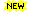Applied Mathematics Numerical Analysis and Scientific Computing Electronic Engineering Computational and Algorithmic Mathematics
•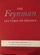The Feynman Lectures on Physics (Richard P. Feynman, et al.)

Ranging from the most basic principles of Newtonian physics through such formidable theories as general relativity and quantum mechanics, Feynman's lectures stand as a monument of clear exposition and deep insight.

•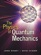The Physics of Quantum Mechanics (James Binney, et al.)

This book aims to give students a good understanding of how quantum mechanics describes the material world. It shows that the theory follows naturally from the use of probability amplitudes to derive probabilities.

•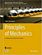Principles of Mechanics: Fundamental University Physics

This textbook takes the reader step-by-step through the concepts of mechanics in a clear and detailed manner. Many proofs and examples are included to help the reader grasp the fundamentals fully, paving the way to deal with more advanced topics.

•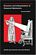Structure and Interpretation of Classical Mechanics

This innovative textbook concentrates on developing general methods for studying the behavior of classical systems. It focuses on the phenomenon of motion and makes extensive use of computer simulation in its explorations of the topic.

•Variational Principles in Classical Mechanics (Douglas Cline)

This book introduces variational principles and their application to classical mechanics. The relative merits of the intuitive Newtonian vectorial formulation, and the more powerful variational formulations are compared.

•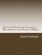Lecture Notes on Classical Mechanics (Sunil Golwala)

You will learn a variety of new techniques and formalism that will allow you to attack a wider set of problems than you saw in the introductory sequences, more unified understanding of the structure and fundamental principles of classical physics.

•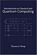Introduction to Classical and Quantum Computing (Tom Wong)

This book is for students who want to learn quantum computing beyond a conceptual level, but who lack advanced training in mathematics. The only prerequisite is trigonometry, and mathematics beyond that will be covered.

•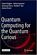Quantum Computing for the Quantum Curious

This open access book makes quantum computing more accessible than ever before. It bridges the gap between popular science articles and advanced textbooks by making key ideas accessible with just high school physics as a prerequisite.

•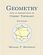Geometry with an Introduction to Cosmic Topology

The text uses Mobius transformations in the extended complex plane to define and investigate these three candidate geometries, thereby providing a natural setting in which to express results common to them all, as well as results that encapsulate key differences.

•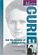Marie Curie and the Science of Radioactivity (Naomi Pasachoff)

Marie Curie discovered radium and went on to lead the scientific community in studying the theory behind and the uses of radioactivity. She left a vast legacy to future scientists through her research, her teaching, and her contributions to the welfare of humankind.

•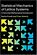Statistical Mechanics of Lattice Systems: Mathematical Introduction

This motivating textbook gives a friendly, rigorous introduction to fundamental concepts in equilibrium statistical mechanics, covering a selection of specific models, including the Curie–Weiss and Ising models, the Gaussian free field, etc.

•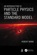An Introduction to Particle Physics and the Standard Model

This book familiarizes readers with what is considered tested and accepted and in so doing, gives them a grounding in particle physics in general, takes an historical approach showing how the model is linked to the physics in less challenging areas.

•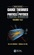Gauge Theories in Particle Physics: A Practical Introduction

Offers an accessible and practical introduction to the three gauge theories in the Standard Model of particle physics: quantum electrodynamics (QED), quantum chromodynamics (QCD), and the Glashow-Salam-Weinberg (GSW) electroweak theory.

•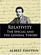Relativity: The Special and General Theory (Albert Einstein)

The great physicist himself disclaimed this exclusionary view, and in this book, he explains both theories in their simplest and most intelligible form for the layman not versed in the mathematical foundations of theoretical physics.

•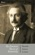The Einstein Theory of Relativity (Hendrik A. Lorentz)

The books published on the subject are so technical that only a person trained in pure physics and higher mathematics is able to fully understand them. In order to make a popular explanation of this far-reaching theory available, the present book is published.

•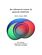An Advanced Course in General Relativity (Eric Poisson)

Focusing on conceptual clarity, he derives all the basic results in the simplest way, taking care to explain the physical, philosophical and mathematical ideas at the heart of "the most beautiful of all scientific theories".

•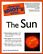Complete Idiot's Guide to the Sun (Jay M. Pasachoff)

Everything revolves around it...and now skywatchers and armchair scientists can learn all about the origin and history of the sun. With information on the sun's physical properties; how solar flares, sunspots, ...

•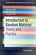Introduction to Random Matrices: Theory and Practice

This is a book for absolute beginners. The aim is to provide a truly accessible introductory account of Random Matrix theory. Most chapters are accompanied by Matlab codes to guide readers through the numerical check of most analytical results.

•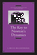The Key to Newton's Dynamics (J. Bruce Brackenridge)

This book clearly explains the surprisingly simple analytical structure that underlies the determination of the force necessary to maintain ideal planetary motion, sets the problem in historical and conceptual perspective, showing the works of both Descartes and Galileo.

•Learn Quantum Computation using Qiskit

This is a free digital textbook that will teach you the concepts of quantum computing while you learn to use the Qiskit SDK. It is built on a jupyter notebook framework that allows for easy reading, but it also allows readers to edit and run the code right in the textbook.

•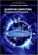Quantum Computing: Progress and Prospects

Provides an introduction to the Quantum Computing, including the unique characteristics and constraints of the technology, and assesses the feasibility and implications of creating a functional quantum computer capable of addressing real-world problems.

•Quantum Computing Since Democritus (Scott Aaronson)

This book takes readers on a tour through some of the deepest ideas of maths, computer science and physics. Full of insights, arguments and philosophical perspectives, the book covers an amazing array of topics.

•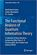The Functional Analysis of Quantum Information Theory

This book provides readers with a concise introduction to current studies on operator-algebras and their generalizations, operator spaces and operator systems, with a special focus on their application in Quantum Information Science.

•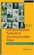Computational Turbulent Incompressible Flow: Applied Math

This book presents a unified new approach to computational simulation of turbulent flow starting from the general basis of calculus and linear algebra. It puts the Body and Soul computational finite element methodology in practical use.

•Applied Computational Fluid Dynamics (Hyoung Woo Oh)

This book is served as a reference text to meet the needs of advanced scientists and research engineers who seek for their own computational fluid dynamics (CFD) skills to solve a variety of fluid flow problems.

•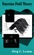Bayesian Field Theory (Jorg C. Lemm)

Long the province of mathematicians and statisticians, Bayesian methods are applied in this ground-breaking book to problems in cutting-edge physics, with practical examples of Bayesian analysis for the physicist working in such areas as neural networks, artificial intelligence, and inverse problems in quantum theory.

•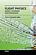Flight Physics - Models, Techniques and Technologies (K. Volkov)

Focuses on the synthesis of the fundamental disciplines and practical applications involved in the investigation, description, and analysis of aircraft flight including applied aerodynamics, aircraft propulsion, flight performance, stability, and control.

•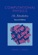Computational Physics: An Introduction (Franz J. Vesely)

A concise introduction to the methods and algorithms used in computational physics, clear in its presentation, useful for those beginning more advanced work in the field. Sample programs are be written in JAVA and are accompanied by short explanations.

•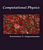Computational Physics and Scientific Computing: C++ or Fortran

This book is an introduction to the computational methods used in physics, but also in other scientific fields. Both C++ or Fortran are used for programming the core programs and data analysis is performed using the powerful tools of the Gnu/Linux environment.

•Computational Physics with Python (Eric Ayars)

This book provides an unusually broad survey of the topics of modern computational physics. Its philosophy is rooted in learning by doing, with new scientific materials as well as with the Python programming language.

•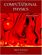Computational Physics with Python (Mark Newman)

A complete introduction to the field of computational physics, with examples and exercises in the Python programming language. It explains the fundamentals of computational physics and describes in simple terms the techniques that every physicist should know,.

•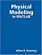Physical Modeling in MATLAB (Allen B. Downey)

Written for beginners, this book provides an introduction to programming in MATLAB and simulation of physical systems. Most books that use MATLAB are aimed at readers who know how to program. This book is for people who have never programmed before.

•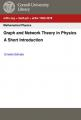Graph and Network Theory in Physics: A Short Introduction

It consists of some of the main areas of research in graph and network theory applied to physics, includes graphs in condensed matter theory, such as the tight-binding and the Hubbard model and statistical physics by means of the analysis of the Potts model ...

•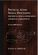Physical Audio Signal Processing (Julius O. Smith III)

This book describes signal-processing models and methods that are used in constructing virtual musical instruments and audio effects. The goal of this book is to enable the reader in developing virtual musical instruments and audio effects that can be boiled down to algorithms and executed by a computer.

•Numerical Simulations of Physical and Engineering Processes

The book handles the numerical simulations of physical and engineering systems. It can be treated as a bridge linking various numerical approaches of two closely inter-related branches of science, i.e. physics and engineering.

•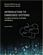Introduction to Embedded Systems - A Cyber-Physical Systems

This book strives to identify and introduce the durable intellectual ideas of embedded systems as a technology and as a subject of study. It emphasizes on modeling, design, and analysis of cyber-physical systems, which integrate computing, networking, etc.

•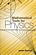Mathematical Tools for Physics (James Nearing)

This book helps physics students learn to take an informed and intuitive approach to solving problems. It assists undergraduates in developing their skills and provides them with grounding in important mathematical methods.

•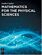Mathematics for the Physical Sciences (Leslie Copley)

This book provides a comprehensive introduction to the areas of mathematical physics. It combines all the essential math concepts into clearly written reference and illustrates the mathematics with numerous physical examples drawn from contemporary research.

•Mathematics for the Physical Sciences (Herbert S. Wilf)

This book provides a text for a first-year graduate level course in mathematical methods. Advanced undergraduates and graduate students in the natural sciences will receive a solid foundation in several fields of mathematics with this text.

•Mathematical Foundations of Quantum Theory

This is a collection of lecture notes, all shared a common interest in answering quantum issues. The goal is to give a mathematically clear and self-containing explanation of the main concepts of the modern language of quantum theory.

•Linear Algebra: A Course for Physicists and Engineers (Arak Mathai)

This textbook on linear algebra is written to be easy to digest by non-mathematicians. It introduces the concepts of vector spaces and mappings between them without too much theorems and proofs. Various applications of the formal theory are discussed as well.

•Probability and Statistics: A Course for Physicists and Engineers

It offers an introduction to concepts of probability theory, probability distributions relevant in the applied sciences, and focuses on real engineering applications, as well as basics of sampling distributions, estimation and hypothesis testing.

•Theory of Seismic Imaging (John A. Scales)

This book presents the physical and mathematical basis of imaging algorithms in the context of controlled-source reflection seismology. The approach taken is motivated by physical optics and theoretical seismology.

•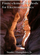Finite-element Methods for Electromagnetics (Stanley Humphries)

This book covers a broad range of practical applications involving electric and magnetic fields. The text emphasizes finite-element techniques to solve real-world problems in research and industry.

•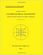Combinatorial Geometry with Application to Field Theory

Topics covered in this book include fundamental of combinatorics, algebraic combinatorics, topology with Smarandache geometry, combinatorial differential geometry, combinatorial Riemannian submanifolds, Lie multi-groups, etc.

•Python Scripting for Computational Science (Hans Langtangen)

With a primary focus on examples and applications of relevance to computational scientists, this brilliantly useful book shows computational scientists how to develop tailored, flexible, and human-efficient working environments built from small scripts written in the easy-to-learn, high-level Python language.

•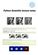Python Scientific Lecture Notes (Scipy Lectures )

This book is the teaching material on the scientific Python ecosystem, a quick introduction to central tools and techniques. It is for programmers from beginner to expert. Work on real-world problems with SciPy, NumPy, Pandas, scikit-image, and other Python libraries.

•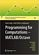Programming for Computations - MATLAB/Octave (Svein Linge)

This book presents computer programming as a key method for solving mathematical problems using MATLAB and Octave. It is intended for novice programmers. Each treated concept is illustrated and explained in detail by means of working examples.

•Programming for Computations - Python (Svein Linge, et al)

This book presents computer programming as a key method for solving mathematical problems using Python. Each treated concept is illustrated and explained in detail by means of working examples. It is intended for novice programmers and engineers.

•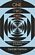One Two Three ... Infinity: Facts and Speculations of Science

Whatever your level of scientific expertise, chances are you'll derive a great deal of pleasure, stimulation, and information from this unusual and imaginative book. It belongs in the library of anyone curious about the wonders of the scientific universe.

•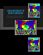Linear Mathematics In Infinite Dimensions (Ulrich H. Gerlach)

Focus on the mathematical framework that underlies linear systems arising in physics, engineering and applied mathematics - from the theory of linear transformation on finite dimensional vector space to the infinite dimensional vector spaces.

•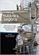Hubble's Legacy (Roger D. Launius, et al)

This book, which includes contributions from historians of science, key scientists and administrators, and one of the principal astronauts who led many of the servicing missions, is meant to capture the history of this iconic instrument.

•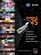Hubble 25: A Quarter-Century of Discovery with the Telescope

In celebration of the Hubble Space Telescope's 25th anniversary, explore 25 of Hubble's breathtaking and significant images. Along with companion descriptions and videos, the 25 images highlight the telescope's amazing capabilities.

•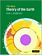New Theory of the Earth (Don L. Anderson)

This interdisciplinary advanced textbook on the origin, composition, and evolution of Earth's interior provides a complete update of Anderson's Theory of the Earth, including many new sections, figures and tables.

•Python in Hydrology (Sat Kumar Tomer)

This book is written for learning Python using its applications in hydrology. The book covers the basic applications of hydrology, and also the advanced topic like use of copula.

•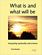What is and What will be: Integrating Spirituality and Science

This book integrates science with values and meaning by making the simplest possible assumption about the connection between physical structure and conscious experience. This follows from Gödel's Incompleteness Theorem and the assumptions of this book.

Book Categories
 :All CategoriesTop Free BooksRecent BooksMiscellaneous BooksComputer EngineeringComputer LanguagesComputer ScienceData Science/DatabasesJava and Java EE (J2EE)Linux and UnixMathematicsMicrosoft and .NETMobile ComputingNetworking and CommunicationsSoftware EngineeringSpecial TopicsWeb Programming
Other Categories